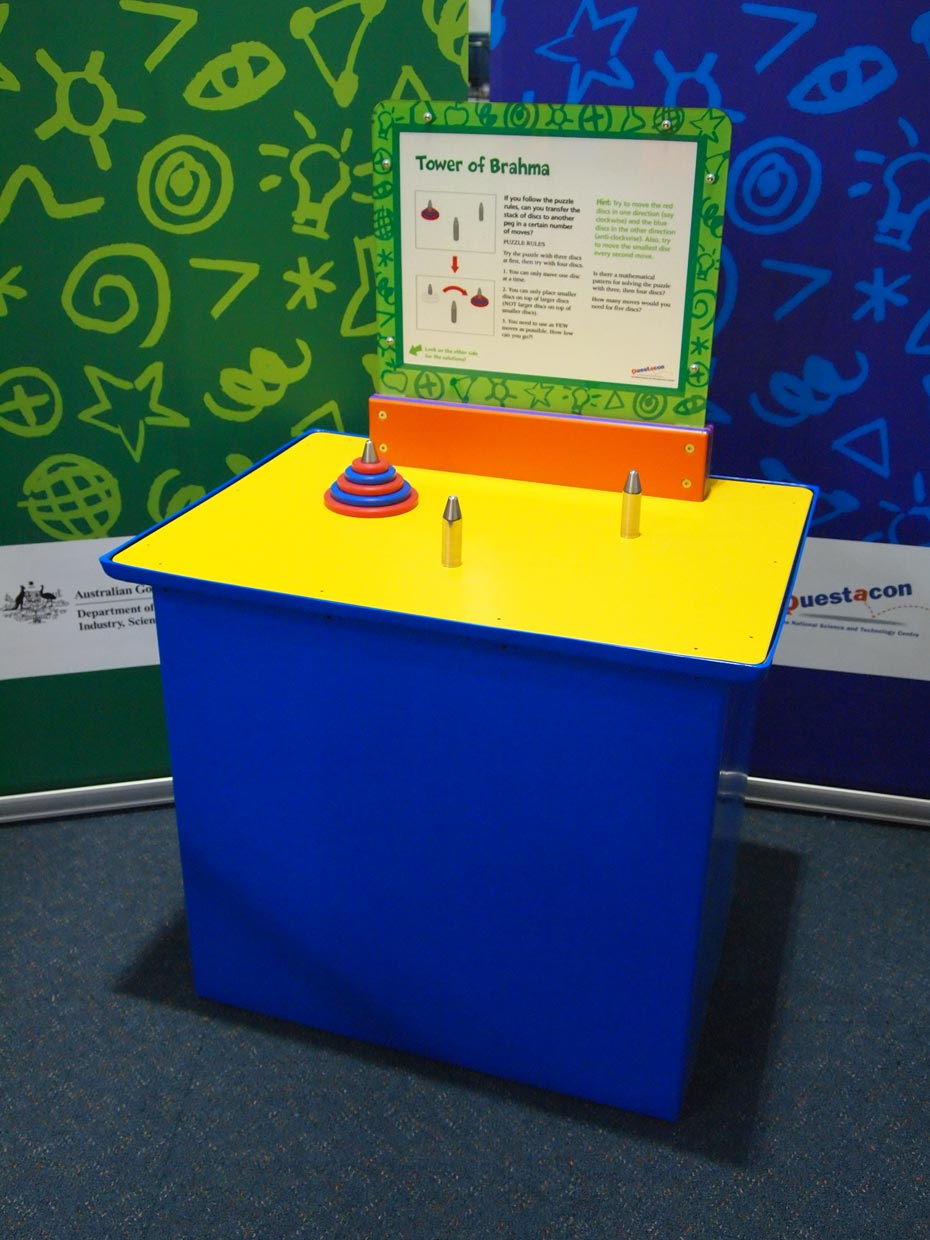# Tower of BrahmaThe minimum number of moves required to solve a Tower of Brahma puzzle can be calculated according to the number of puzzle pieces.

## How it works

Move all discs from one peg to another peg, using certain rules and within a minimum number of moves.

## Things to try or ask around the exhibit

• If you follow the puzzle rules, can you transfer the stack of discs to another peg in a certain number of moves?
• Is there a mathematical pattern for solving the puzzle with three, then four discs?
• How many moves would you need for five discs?

## Background

When you solve the Tower of Brahma puzzle, you need at least:

• 7 moves for 3 discs
• 15 moves for 4 discs
• moves for 5 discs.

The solution can be represented by a mathematical formula, where n equals the number of discs and the minimum number of moves needed to solve the puzzle is 2n - 1. This formula used to calculate the least number of moves is known as a Recurrence Relation.

## Finding the science in your world

Psychologists sometimes use the Tower of Brahma puzzle as a test for dementia and problem-solving abilities, because the problem is relatively simple and steps in the solution can be traced and recorded.